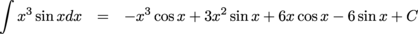Study Guide

# Indefinite Integrals - In the Real World

## In the Real World

We'll be honest: a lot of the mechanical integration methods you're learning here probably won't be that useful in the long run. Once you get out of school and into a real-life situation, you'll get to use computers most of the time.

The most useful aspect of the integration problems isn't the integration. It's the practice you get at figuring out how to attack each new problem and which integration technique to use. This will make you generally better at figuring out what to do when you encounter new types of problems.

The improper integrals are more useful. Improper integrals play a large role in the study of probability, once we get beyond problems like "what is the likelihood of picking a blue sock out of the drawer?" Improper integrals are also used to create the Fourier transform and the Laplace transform, which physicists and engineers use to help solve certain types of differential equations.

• ### I Like Abstract Stuff; Why Should I Care?

Limits are something the human race (at least, the mathematicians) have created as a result of trying to find a way to think about infinity.

Another way of thinking about infinity involves things called infinitesimals. An infinitesimal is a number that's bigger than 0, but smaller than all normal everyday fractions. If ε is an infinitestimal, then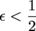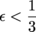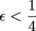...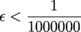...

and yet,

0 < ε.

This website has a great discussion of limits and infinitesimals.

• ### How to Solve a Math Problem

There are three steps to solving a math problem.

1. Figure out what the problem is asking.

2. Solve the problem.

### Sample Problem

Find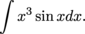1. Figure out what the problem is asking.

The problem is asking us to find the family of all functions with derivative x3 sin x. That means we need to use some of our integration techniques.

2. Solve the problem.

We can't use substitution, we can't use partial fractions, and we certainly can't just think backwards from this. That leaves integration by parts.

We want u' to be simpler than u, so take

u = x3

v' = sin x.

Then

u' = 3x2

v = -cos x

Apply the magic formula:To find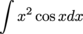we need to use integration by parts again. We still want u' to be simpler than u, so take

u = x2

v' = cos x

u' = 2x

v = sin x

Apply the magic formula:To find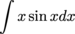we need to use integration by parts yet again. Take

u = x

v' = sin x

u' = 1

v = -cos x

Then apply the formula again:Now we can go up a step and finish our second integration by parts.Finally we go back up to the first integration by parts: